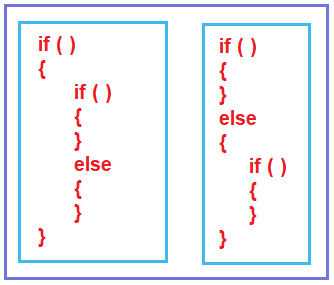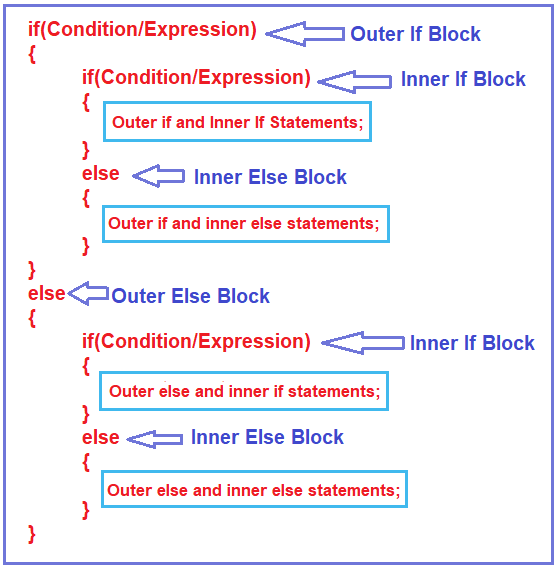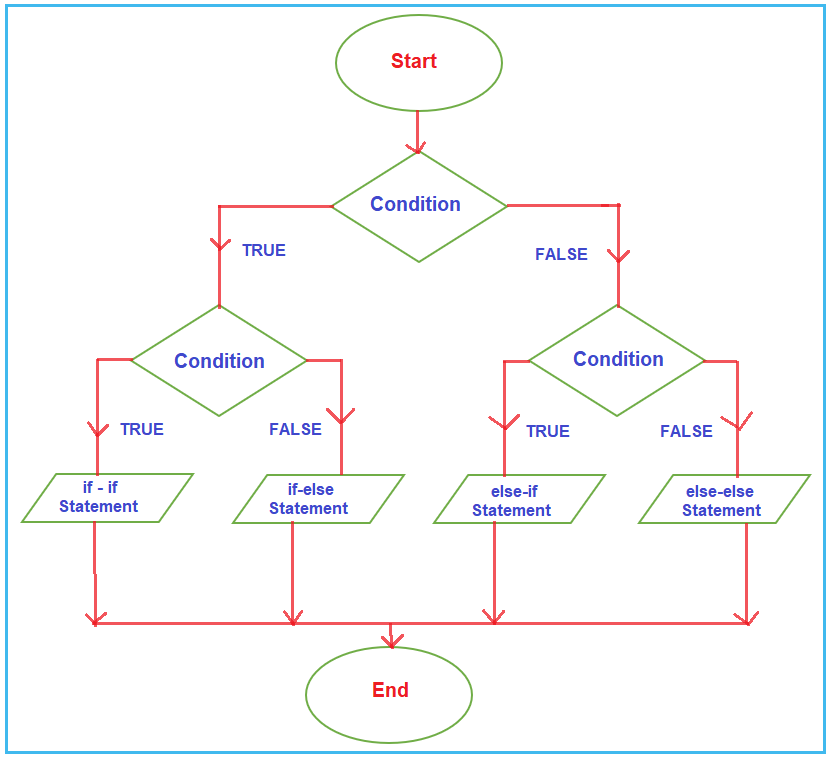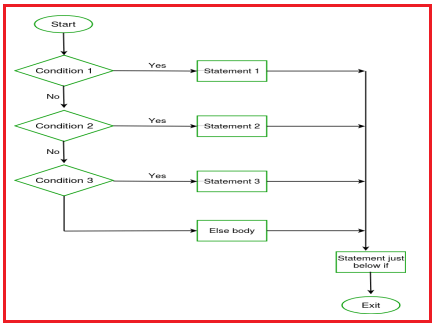# Nested If-Else Statement in C

## Nested If-Else Statement in C Language with Examples

In this article, we will discuss Nested If-Else Statement in C Language with Syntax, Flowchart, and Examples. Please read our previous article, where we discussed the if-else conditional statement in C Language with examples.

##### Nested if-else statements in C Language:

In the C programming language, nested if-else statements are a way to check multiple conditions and make decisions based on those conditions. The concept involves placing an if-else block inside another if-or-else block. This is useful for handling complex decision-making scenarios.

When an if-else statement is present inside the body of another if or else, this is called nested if-else. Nested if statements are used when we want to check for a condition only when a previous dependent condition is true or false. C allows us to nested if statements within if statements, i.e., we can place an if statement inside another if statement.

##### What is the Nested If block?

Nested if block means defining an if block inside another if block. We can also define the if block inside the else blocks. Depending on our requirements, we can use nested if block in n number of ways. You can define nested if blocks at many levels. First, we will see the syntax and example, and in the later part of this article, we will understand the flowchart by taking one example.

##### Nested If-Else Statement Syntax in C Language:

Please have a look at the image below, which shows the different ways to use the nested if block in the C Programming Language.We will take one example and try to understand the flow chart. We are taking the following syntax. Here, we have an if-else block inside the if block, as well as an if-else block inside the else block.##### How Does Nested IF ELSE Work in C Language?

First, it will check the first if condition, i.e., the outer if condition, and if it is true, then the outer else block will be terminated. So, the control moves inside the first or the outer if block. Then again it checks the inner if condition, and if the inner if condition is true, the inner else block is terminated. So, in this case, the outer if and inner if block statements get executed.

Now, if the outer if condition is true, but the inner if condition is false, the inner if block gets terminated. So, in this case, the outer if and inner else block statements get executed.

Now, if the outer if condition is false, then the outer if block gets terminated, and control moves to the outer else block. And inside the outer else block, again, it checks the inner if condition, and if the inner if condition is true, the inner else block gets terminated. So, in this case, the outer else and inner if block statements get executed.

Now, if the outer if condition is false, as well as the if condition inside the outer else blocks also failed, then the if block gets terminated. In this case, the outer else and inner else block statements get executed. This is how statements get executed in Nested if. Now, we will see the flow chart of nested if blocks.

##### Flow Chart of Nested If Block in C Programming Language:

First, have a look at the diagram below, which shows the flow chart of the nested if-else statement.First, it will check the outer if condition, and if the outer if condition is true, then the control comes inside and it will check the inner if condition, and if the inner if condition is true, then the outer if block statements and inner if block statements get executed. And after execution, it will come to an end.

Suppose the outer if condition is true, but the inner if condition fails. Then, the outer if block statements and the inner else block statement get executed. And once the statement gets executed, it will come to an end.

Suppose the outer if condition fails; then the control directly comes to the else block and checks the inner if condition. And again, for the inner if condition, two options are there. If the inner if condition is true, then it will execute the outer else block and inner if block statement, and if the inner if condition is false, then it will execute the outer else block and inner else block statements and then come to an end.

##### Program to Understand Nested IF-ELSE Statements in C Language:
```#include <stdio.h>
int main()
{
int i = 10;
if (i == 10)
{
if (i < 15) // First if statement
printf("i is smaller than 15\n"); // Nested - if statement
// Will only be executed if statement above is true.
if (i < 12)
printf("i is smaller than 12 too\n");
else
printf("i is greater than 15");
}
return 0;
}```
###### Output:##### Ladder if-else statements in C Language:

In Ladder if-else statements, one of the statements will be executed depending upon the truth or falsity of the conditions. if condition 1 is true, then Statement 1 will be executed, and so on, but if all conditions are false, then Statement 3 will be executed. The C if statements are executed from the top down. As soon as one of the conditions controlling the if is true, the statement associated with that if is executed, and the rest of the C else-if ladder is bypassed. If none of the conditions are true, then the final else statement will be executed.###### Syntax to use Ladder if-else statements in C Language:##### Program to Understand Ladder if-else statements in C Language:
```#include <stdio.h>
int main()
{
int i = 20;
if (i == 10)
{
printf("i is 10");
}
else if (i == 15)
{
printf("i is 15");
}
else if (i == 20)
{
printf("i is 20");
}
else
{
printf("i is not present");
}
}```

Output: i is 20

##### Example: Checker Whether Eligible to Drive

In this example, the program first checks if the age is 18 or older. If this is true, it checks whether the individual has a driving license. Based on these conditions, it decides which message to print.

```#include <stdio.h>

int main() {
int age = 20;
int hasLicense = 1; // let's say 1 means 'yes' and 0 means 'no'

if (age >= 18) {